# How do you write a intersection(∩) in latex?

One of the most important operations in the set is the intersection. Mathematically, you need to use the ∩ symbol to represent this intersection operation.

Properties Value
Symbol Union
Argument No
Command \cap
Example \cap → ∩

As you may know, the \cup command is used to represent union symbols using latex. In the same way, you need to use the \cap command to represent the intersection symbol.

\documentclass{article}
\begin{document}
$s_{1} \cap s_{2}$
\end{document}

Output :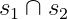You may have noticed that the Big Intersection symbol is used in many science documents.

\documentclass{article}
\begin{document}
$s_{1} \bigcap s_{2}$
\end{document}

Output :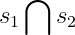In Latex, the default \bigcap command is used to denote this Big Union symbol.

## Latex intersection symbol with latex

When the intersection operation is performed in the n number set. In that case, limits are used above and below the Big Intersection symbol as a sum.

\documentclass{article}
\begin{document}
$\bigcap\limits_{i=1}^{n}F_{i}$
$\bigcap_{i=1}^{n}A_{i}$
\end{document}

Output :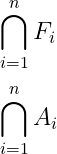If you use the Big Intersection symbol in the text, that is, inline, then the shape of the symbol can be changed.

\documentclass{article}
\begin{document}
latex eqn $\bigcap_{i=1}^{n}x_{i}$
\end{document}

Output :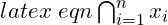If you perform an intersection operation within the disjoint set, it will return an empty set. The \emptyset command is used for this Empty set in Latex.

\documentclass{article}
\begin{document}
$s_{1}\cap s_{2}=\emptyset$
\end{document}

Output :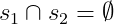Many times intersection operations are performed on infinity number sets. In that case, the upper limit would be infinity.

\documentclass{article}
\begin{document}
$\bigcap_{i=1}^{\infty}A_{i}$
\end{document}

Output :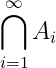You do not need to install any external packages in Latex for all the symbols used above.#### Md Jidan Mondal

LaTeX expert with over 10 years of experience in document preparation and typesetting. Specializes in creating professional documents, reports, and presentations using LaTeX.